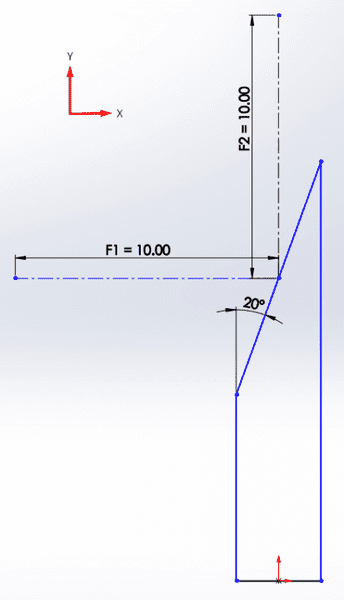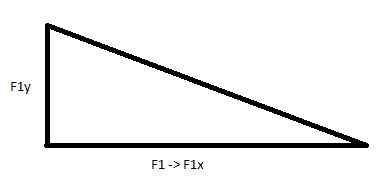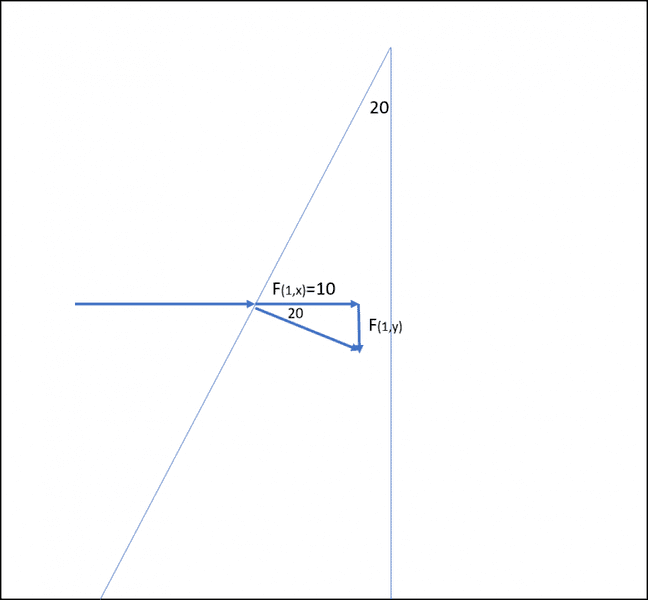# Calculating components of a force applied at an angle

1. The problem statement, all variables, and given/known data
Attached is a diagram of what I am trying to solve. I need to calculate the X and Y components for both F1 and F2. The results will then be used in a later problem that I've already solved but I am coming up with an error and I was unsure about this part of the problem.## The Attempt at a Solution

I tried converting the F1 value into the F1x component and then using trig to solve from that but my number in the later calculation aren't matching up and I think it's coming from this part. This problem yet very similar to the sliding block problem on an incline slope is very different in how things are oriented and set up and it's causing me lots of confusion.Last edited by a moderator:

That diagram is unclear to me without the problem statement itself. Is the blue line supposed to represent an incline that the forces are being applied to that you want to find the next force in the x and y direction for? If so, keep in mind that both F1 and F2 will have both an x and y component acting on the incline that you need to sum.

I really only need to calculate one at a time. They are independent of each other but both forces need to be evaluated. The blue outlined area is a representation of the body the forces are being applied too. All that really needs to be noted is the angle of the inclined plane and the resulting X and Y components aligning with the coordinate system I drew.

Regardless it should be a simple case of trig. Tangent should do it looks like.

Regardless it should be a simple case of trig. Tangent should do it looks like.
Am I doing it correctly in assigning the X component of F1 to F1 itself and then using trig to solve for Y as I have drawn out in the triangle?

It looks off to me. When drawing the diagram, draw the 10 N as a horizontal force into the triangle, draw another coinciding line that is perpendicular to the triangle, and then connect them with a vertical line. If that doesn't make sense, I may be able to draw a picture and upload.Last edited:
haruspex
Homework Helper
Gold Member
2020 Award
Am I doing it correctly in assigning the X component of F1 to F1 itself and then using trig to solve for Y as I have drawn out in the triangle?
If I understand your diagram, F1 is applied in the X direction, so its X component is F1 and its Y component is zero.
Maybe what you intend to ask is the components normal to and parallel to the surface?

It looks off to me. When drawing the diagram, draw the 10 N as a horizontal force into the triangle, draw another coinciding line that is perpendicular to the triangle, and then connect them with a vertical line. If that doesn't make sense, I may be able to draw a picture and upload.

That is what I was thinking. Now I just use trig and solve for F1Y?

If I understand your diagram, F1 is applied in the X direction, so its X component is F1 and its Y component is zero.
Maybe what you intend to ask is the components normal to and parallel to the surface?
That is what I am confused about. If I was pushing against an angled surface with a horizontal force, wouldn't there be resulting X and Y components of forces that can be calculated, like in this sitiation? How I visualised is is how TJGilb drew it. Is that what you're describing as well?

That is what I was thinking. Now I just use trig and solve for F1Y?

Yes.

haruspex